Updating search results...

# 15 Results

View
Selected filters:
• Circumference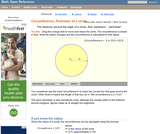Rating
0.0 stars

An interactive applet and associated web page that demonstrate the circumference of a circle. The applet shows a circle with a radius line. The radius endpoints are draggable and the circle is resized accordingly. The formula relating radius to circumference is updated continually as you drag. Introduces the idea of Pi. The formula can be hidden for class discussion and estimation. See also the entries for circumference and diameter. See also entries for radius and diameter. Applet can be enlarged to full screen size for use with a classroom projector. This resource is a component of the Math Open Reference Interactive Geometry textbook project at http://www.mathopenref.com.

Subject:
Geometry
Material Type:
Simulation
Provider:
Math Open Reference
Author:
John Page
02/16/2011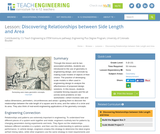Educational Use
Rating
0.0 stars

Through this lesson and its two associated activities, students are introduced to the use of geometry in engineering design, and conclude by making scale models of objects of their choice. The practice of developing scale models is often used in engineering design to analyze the effectiveness of proposed design solutions. In this lesson, students complete fencing (square) and fire pit (circle) word problems on two worksheets—which involves side and radius dimensions, perimeters, circumferences and areas—guiding them to discover the relationships between the side length of a square and its area, and the radius of a circle and its area. They also think of real-world engineering applications of the geometry concepts.

Subject:
Mathematics
Geometry
Measurement and Data
Material Type:
Lesson
Provider:
TeachEngineering
Author:
Aaron Lamplugh
Malinda Zarske
Nathan Coyle
Russell Anderson
Ryan Sullivan
02/07/2017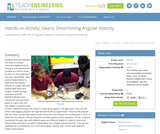Educational Use
Rating
0.0 stars

Students work as engineers and learn to conduct controlled experiments by changing one experimental variable at a time to study its effect on the experiment outcome. Specifically, they conduct experiments to determine the angular velocity for a gear train with varying gear ratios and lengths. Student groups assemble LEGO MINDSTORMS(TM) NXT robots with variously sized gears in a gear train and then design programs using the NXT software to cause the motor to rotate all the gears in the gear train. They use the LEGO data logging program and light sensors to set up experiments. They run the program with the motor and the light sensor at the same time and analyze the resulting plot in order to determine the angular velocity using the provided physics-based equations. Finally, students manipulate the gear train with different gears and different lengths in order to analyze all these factors and figure out which manipulation has a higher angular velocity. They use the equations for circumference of a circle and angular velocity; and convert units between radians and degrees.

Subject:
Engineering
Material Type:
Activity/Lab
Provider:
TeachEngineering
Provider Set:
TeachEngineering
Author:
James Cox
Jasmin Mejias
Jennifer S. Haghpanah
Leonarda Huertas
Mihai Pruna
09/18/2014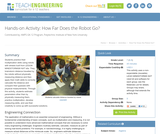Educational Use
Rating
0.0 stars

Students practice their multiplication skills using robots with wheels built from LEGO® MINDSTORMS® NXT kits. They brainstorm distance travelled by the robots without physically measuring distance and then apply their math skills to correctly calculate the distance and compare their guesses with physical measurements. Through this activity, students estimate parameters other than by physically measuring them, practice multiplication, develop measuring skills, and use their creativity to come up with successful solutions.

Subject:
Engineering
Mathematics
Physics
Material Type:
Activity/Lab
Provider:
TeachEngineering
Provider Set:
TeachEngineering
Author:
Elina Mamasheva
Keeshan Williams
09/18/2014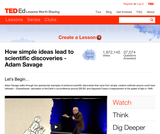Only Sharing Permitted
CC BY-NC-ND
Rating
0.0 stars

Adam Savage walks through two spectacular examples of profound scientific discoveries that came from simple, creative methods anyone could have followed -- Eratosthenes' calculation of the Earth's circumference around 200 BC and Hippolyte Fizeau's measurement of the speed of light in 1849. A quiz, thought provoking question, and links for further study are provided to create a lesson around the 7-minute video. Educators may use the platform to easily "Flip" or create their own lesson for use with their students of any age or level.

Subject:
Physical Science
Material Type:
Lecture
Provider:
TED
Provider Set:
TED-Ed
Author:
Franz Palomares
Jeremiah Dickey
Kari Mullholand
03/13/2012Only Sharing Permitted
CC BY-NC-ND
Rating
0.0 stars

The ratio of a circle's circumference to its diameter is always the same: 3.14159... and on and on (literally!) forever. This irrational number, pi, has an infinite number of digits, so we'll never figure out its exact value no matter how close we seem to get. Reynaldo Lopes explains pi's vast applications to the study of music, financial models, and even the density of the universe.

Subject:
Mathematics
Material Type:
Lecture
Provider:
TED
Provider Set:
TED-Ed
Author:
Reynaldo Lopes
12/27/2013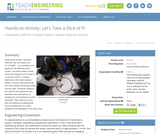Educational Use
Rating
0.0 stars

Working as a team, students discover that the value of pi (3.1415926...) is a constant and applies to all different sized circles. The team builds a basic robot and programs it to travel in a circular motion. A marker attached to the robot chassis draws a circle on the ground as the robot travels the programmed circular path. Students measure the circle's circumference and diameter and calculate pi by dividing the circumference by the diameter. They discover the pi and circumference relationship; the circumference of a circle divided by the diameter is the value of pi.

Subject:
Engineering
Mathematics
Material Type:
Activity/Lab
Provider:
TeachEngineering
Provider Set:
TeachEngineering
Author:
Carole Chen
Michael Hernandez
09/18/2014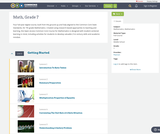Conditional Remix & Share Permitted
CC BY-NC
Rating
0.0 stars

Four full-year digital course, built from the ground up and fully-aligned to the Common Core State Standards, for 7th grade Mathematics. Created using research-based approaches to teaching and learning, the Open Access Common Core Course for Mathematics is designed with student-centered learning in mind, including activities for students to develop valuable 21st century skills and academic mindset.

Subject:
Mathematics
Material Type:
Full Course
Provider:
Pearson
10/06/2016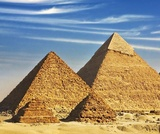Rating
0.0 stars

Zooming In On Figures

Unit Overview

Type of Unit: Concept; Project

Length of Unit: 18 days and 5 days for project

Prior Knowledge

Students should be able to:

Find the area of triangles and special quadrilaterals.
Use nets composed of triangles and rectangles in order to find the surface area of solids.
Find the volume of right rectangular prisms.
Solve proportions.

Lesson Flow

After an initial exploratory lesson that gets students thinking in general about geometry and its application in real-world contexts, the unit is divided into two concept development sections: the first focuses on two-dimensional (2-D) figures and measures, and the second looks at three-dimensional (3-D) figures and measures.
The first set of conceptual lessons looks at 2-D figures and area and length calculations. Students explore finding the area of polygons by deconstructing them into known figures. This exploration will lead to looking at regular polygons and deriving a general formula. The general formula for polygons leads to the formula for the area of a circle. Students will also investigate the ratio of circumference to diameter ( pi ). All of this will be applied toward looking at scale and the way that length and area are affected. All the lessons noted above will feature examples of real-world contexts.
The second set of conceptual development lessons focuses on 3-D figures and surface area and volume calculations. Students will revisit nets to arrive at a general formula for finding the surface area of any right prism. Students will extend their knowledge of area of polygons to surface area calculations as well as a general formula for the volume of any right prism. Students will explore the 3-D surface that results from a plane slicing through a rectangular prism or pyramid. Students will also explore 3-D figures composed of cubes, finding the surface area and volume by looking at 3-D views.
The unit ends with a unit examination and project presentations.

Subject:
Mathematics
Geometry
Material Type:
Unit of Study
Provider:
PearsonConditional Remix & Share Permitted
CC BY-NC
Rating
0.0 stars

Students will measure the circumference and diameter of round things in the classroom and discover the ratio pi. They will see that the ratio of a circle's circumference to its diameter can be used to solve for the circumference when the diameter is known.Key ConceptsStudents have seen circles before, but have not analyzed the relationships between parts of a circle. The ratio of the circumference to the diameter is pi, about 3.14 or about 227. Students see that all of the objects they measure have this ratio (or close, depending on accuracy) and that the ratio is true for all circles. Students also see that the ratio can be used to solve for the circumference of a circle if the diameter (or radius) is known.GoalsMeasure round things looking for similarities.Find the ratio of the circumference to the diameter of those round things.Find a formula to find the circumference of a circle.SWD: Make sure students understand these domain-specific terms:It may be helpful to preteach these terms to students with special needs. If possible, reinforce the definitions of these terms with visual supports (diagrams).ELL: As new vocabulary is introduced, be sure to repeat it several times and to allow students to repeat after you as needed. Write the new words as they are introduced and allow enough time for ELLs to check their dictionaries or briefly consult with another student who shares the same primary language if they wish.ratiocircumferencecirclediameterscatter plot

Subject:
Geometry
Material Type:
Lesson Plan
Provider:
Pearson
09/21/2015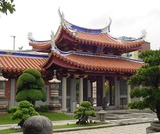Conditional Remix & Share Permitted
CC BY-NC
Rating
0.0 stars

Students critique their work from the Self Check and redo the task after receiving feedback. Students then take a quiz to review the goals of the unit.Key ConceptsStudents understand how to find the area of figures such as rectangles and triangles. They have applied that knowledge to finding the area of composite figures and regular polygons. The area of regular polygons was extended to understand the area of a circle. Students also applied ratio and proportion to interpret scale drawings and redraw them at a different scale.GoalsCritique and revise student work.Apply skills learned in the unit.Understand two-dimensional measurements:Area of composite figures, including regular polygons.Area and circumference of circles.Interpret scale drawings and redraw them at a different scale.SWD: Make sure all students have the prerequisite skills for the activities in this lesson.Students should understand these domain-specific terms:composite figuresregular polygonsareacircumferencescale drawingstwo dimensionalIt may be helpful to preteach these terms to students with disabilities.ELL: As academic vocabulary is reviewed, be sure to repeat it and allow students to repeat after you as needed. Consider writing the words as they are being reviewed. Allow enough time for ELLs to check their dictionaries if they wish.

Subject:
Geometry
Material Type:
Lesson Plan
Provider:
Pearson
09/21/2015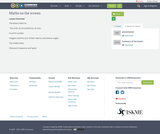Only Sharing Permitted
CC BY-NC-ND
Rating
0.0 stars

The lesson refers to: The circle, its circumference, its areaPi and Fi numberPolygons and the sum of their interior and exterior anglesThe Golden RatioFibonacci’s Sequence and Spiral

Subject:
Computer Science
Graphic Design
Geometry
Ratios and Proportions
Material Type:
Lesson Plan
Author:
Maria Szklarczyk
01/15/2019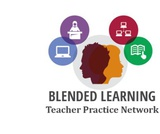Conditional Remix & Share Permitted
CC BY-NC
Rating
0.0 stars

This problem-based learning module is designed to engage students in solving a real problem within the community.  The question being “How can I help my community get digitally connected?”  Students will choose to investigate one of three solutions of making wifi available in our school district to the most populated areas. They will either choose to put Wifi on bus, placing hotspots in the community or using kajeet.   The students will be using Google Earth Pro to place circles on a map and calculating the area of these circles.  Students will make a model of these circles onto a hard copy using scale factor. At the conclusion, the students will present findings to administration, the board of education, state and local leaders as well as their peers.  These findings can be presented through the choice of a display board, flyer, video production or prezi.This blended module includes teacher-led discussion, group-led investigation and discussions along with technology integration.

Subject:
Mathematics
Geometry
Material Type:
Lesson Plan
Author:
Blended Learning Teacher Practice Network
11/21/2017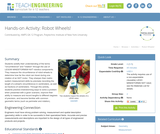Educational Use
Rating
0.0 stars

Students solidify their understanding of the terms "circumference" and "rotation" through the use of LEGO MINDSTORMS(TM) NXT robotics components. They measure the circumference of robot wheels to determine how far the robot can travel during one rotation of an NXT motor. They sharpen their metric system measurement skills by precisely recording the length of a wheel's circumference in centimeters, as well as fractions of centimeters. Through this activity, students practice brainstorming ways to solve a problem when presented with a given scenario, improve their ability to measure and record lengths to different degrees of precision, and become familiar with common geometric terms (such as perimeter and rotation).

Subject:
Engineering
Electronic Technology
Material Type:
Activity/Lab
Provider:
TeachEngineering
Provider Set:
TeachEngineering
Author:
Ursula Koniges
09/18/2014Educational Use
Rating
0.0 stars

Students learn that math is important in navigation and engineering. They learn about triangles and how they can help determine distances. Ancient land and sea navigators started with the most basic of navigation equations (speed x time = distance). Today, navigational satellites use equations that take into account the relative effects of space and time. However, even these high-tech wonders cannot be built without pure and simple math concepts â basic geometry and trigonometry â that have been used for thousands of years.

Subject:
Education
Material Type:
Activity/Lab
Provider:
TeachEngineering
Provider Set:
TeachEngineering
Author:
Janet Yowell
Jeff White
Malinda Schaefer Zarske
Matt Lippis